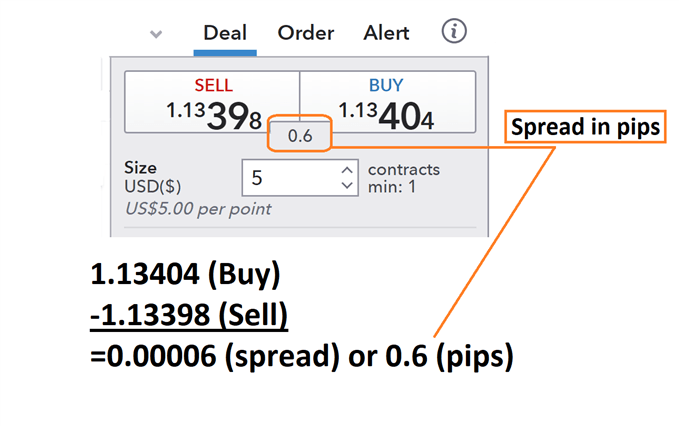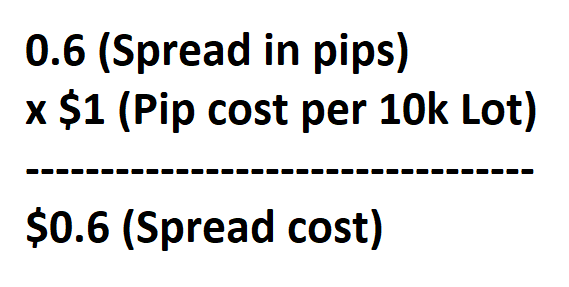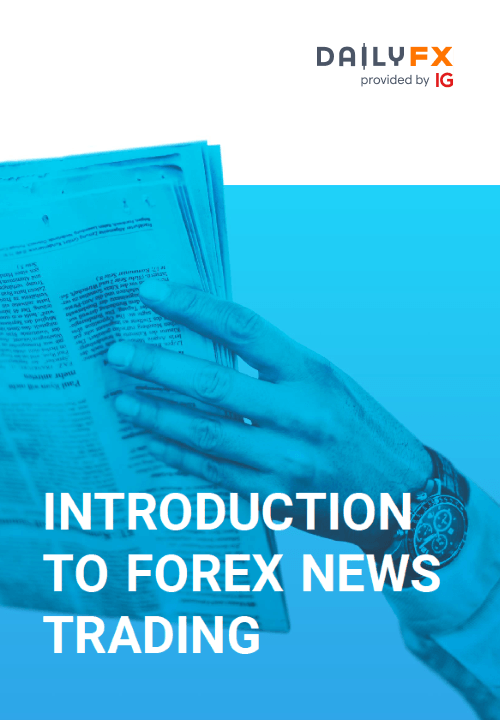Forex spreads explained: Main talking points

• Spreads are based on the buy and sell price of a currency pair.
• Costs are based on forex spreads and lot sizes.

Every market has a spread and so does forex. A spread is simply defined as the price difference between where a trader may purchase or sell an underlying asset. Traders that are familiar with equities will synonymously call this the Bid: Ask spread.

Below we can see an example of the forex spread being calculated for the EUR/USD. First, we will find the buy price at 1.13398 and then subtract the sell price of 1.3404. What we are left with after this process is a reading of .00006. Traders should remember that the pip value is then identified on the EUR/USD as the 4th digit after the decimal, making the final spread calculated as 0.6 pips.Now we know how to calculate the spread in pips, let’s look at the actual cost incurred by traders.

## How to calculate the forex spread and costs

Before we calculate the cost of a spread, remember that the spread is just the ask price less (minus) the bid price of a currency pair. So, in our example above, 1.13404-1.13398 = 0.00006 or 0.6 pips.

Using the quotes above, we know we can currently buy the EUR/USD at 1.13404 and close the transaction at a sell price of 1.13398. That means as soon as our trade is open, a trader would incur 0.6 pips of spread.

To find the total spread cost, we will now need to multiply this value by pip cost while considering the total amount of lots traded. When trading a 10k EUR/USD lot, you would incur a total cost of 0.00006 (0.6pips) X 10,000 (10k lot) = \$0.6. If you were trading a standard lot (100,000 units of currency) your spread cost would be 0.00006pips (0.6pips) X 100,000 (1 standard lot) = \$6.

If your account is denominated in another currency, like GBP, you would have to convert it to US Dollars.It’s important to note that the FX spread can vary over the course of the day, ranging between a ‘high spread’ and a ‘low spread’.

This is because the spread can be influenced by multiple factors like volatility or liquidity. You will notice that some currency pairs, like emerging market currency pairs, have a greater spread than major currency pairs. Your major currency pairs trade in higher volumes compared to emerging market currencies, and higher trade volumes tend to lead to lower spreads under normal conditions.

Additionally, it’s well known that liquidity can dry up and spreads can widen in the lead up to major news events and in between trading sessions.